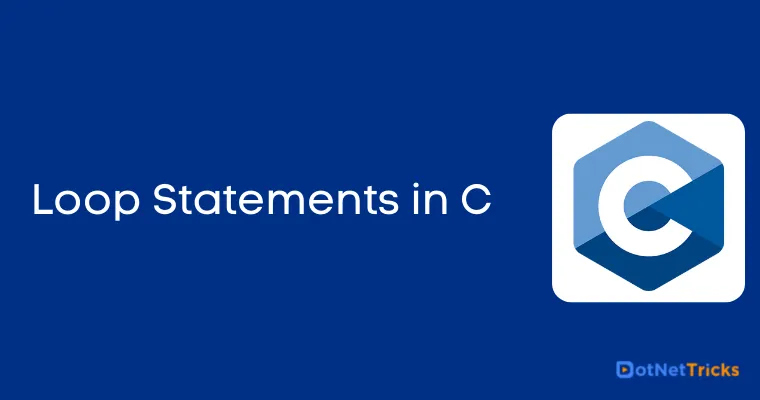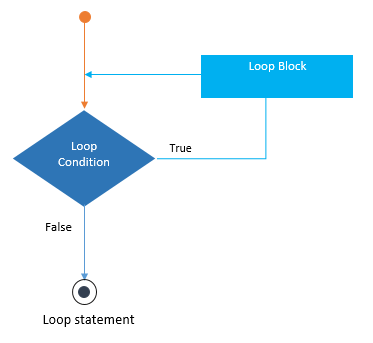# Loop Statements in C

21 Aug 2022
Intermediate
13.9K Views

Loops are used to repeat a block of statements for a certain time. C language offers three types of loops - while, do-while and for loops for iterating a set of statements.

## While

This loop executes a statement or a block of statements until the specified boolean expression evaluates to false.```#include<stdio.h>
#include<conio.h>
void main()
{
clrscr();
int number = 5;
while (number != 0) //specified boolean expression
{
printf("Current value of n is %d", number);
number--; //update statement
}
getch();
}
/* Output:
Current value of n is 5
Current value of n is 4
Current value of n is 3
Current value of n is 2
Current value of n is 1
*/
```

#### Important Note

Typically, while loop is useful when you are not aware of exact number of iterations.

## Do-While Loop

This loop executes a statement or a block of statements until the specified boolean expression evaluates to false.

```#include<stdio.h>
#include<conio.h>
void main()
{
clrscr();
char choice = 'Y';
do
{
int number = 5;

printf("\nPrint Number : %d", number);

printf("\nDo you want to continue (y/n) :");
scanf("%c",&choice);

} while (choice == 'y'); //specified boolean expression

getch();
}
/*
Output:
Print Number : 5
Do you want to continue (Y/N) :y

Print Number : 5
Do you want to continue (Y/N) :n
*/
```

#### Important Note

Typically, while loop is useful when you are not aware of exact number of iterations.

## For Loop

This loop has three sections - index declaration, condition (boolean expression) and updation section. In each for loop iteration, the index is updated (incremented/decremented) by updating section and checked with the condition. If the condition is matched, it continues execution until the specified boolean expression evaluates to false.

```#include<stdio.h>
#include<conio.h>
int main()
{
clrscr();
int a,f,i;
printf("Enter a number: ");
scanf("%d",&a);
f=1;
for(i=1;i<=a;i++)
f = f * i;
printf("Factorial: %d",f);
getch();
}
/*
Output:
Enter a number:5
Factorial:120
Enter a number:4
Factorial:24
*/
```

#### Important Note

Typically, for loop is useful when you are aware of exact number of iterations. Normally, it is used for iterating over arrays and for sequential processing.

### While, Do..While and For Loop

A program can be build by using one of the loop statement. For example, we can print numbers from 1 to 100 using all loops.

While Loop example
```int i=0;
while(i<100)
{
i++;
printf("%d\n", i);
}
```
Do While Loop example
``` int i=0;
do
{
i++;
printf("%d\n",i);
}while(i<100);
```
For Loop example
``` for(int i = 1; i <= 100; i++)
{
printf("%d\n", i);
}
```

Learn to Crack Your Technical Interview